### Home > CALC > Chapter 6 > Lesson 6.1.2 > Problem6-28

6-28.
1. Since y = ex and y = ln x are inverse functions, eln x = x and ln ex = x. Use these facts along with exponent and log laws to rewrite each expression. Homework Help ✎

1. e2 ln x

2.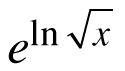3.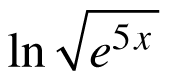4. ln(5ex )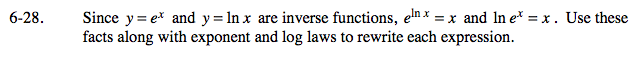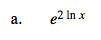e2lnx = elnx2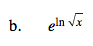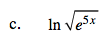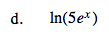ln(5ex) = ln(eln5ex)